Next: Optimization Up: Summary of problems and Previous: Algebraic method

### Jacobian inversion

One of the problems associated with the Jacobian inversion method is the matrix inversion. The Jacobian matrix dimension grows with the degree of freedom. The computation cost of the matrix inversion is growing quite fast with the dimension. Therefore, this method becomes consuming a lot of time for articulated structures with a large number of DOF (e.g. snake, chain, etc.).

In the case when the degree of freedom is different than 6 in 3D space (3 for location, 3 for rotation) -- generally, if the dimension of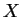is not equal to the dimension of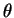, the Jacobian matrix is rectangular and consequently not invertible. In that case, pseudo-inversion could be used for the rectangular matrix inversion by singular value decomposition [GK65]. The disadvantage of the pseudo-inversion is that some numerical errors appears, because that method is approximate and local. If the change ofis too large, according to the facts mentioned above, errors often occur. These errors are called tracking errors'' [WW92]. The solution of that problem is to divide the path into smaller steps.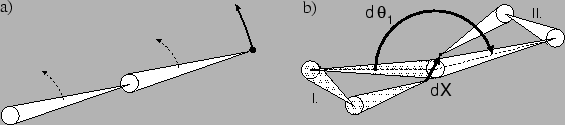When the rank of the Jacobian matrix differs from the DOFs (some rows are linearly dependent), the number of solutions is infinite and the matrix is not invertible. Singularities usually occur in the full extended state (Figure 5a -- the change of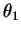and/or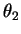causes the same differential movements of the end effector). There is not just a single solution in that situation, a choice has to be done, or the prevention of such states (e.g. used in robotics - singular states are not allowed).

If the structure is near the singular state or at the transition between singular and nonsingular state, high velocities and oscillations occur. Example of such a problematic state is shown in Figure 5b. In the example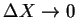,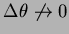. It means that the distance in Cartesian space is small, but the distance in joint space is large (compared to the Cartesian space). That state is called ill conditioned'' [WW92].

The good feature of this method is its expected behavior. The articulated structure behaves like rubber. This method is also quite fast for smaller articulated structures therefore it could be used in the real time animations.Next: Optimization Up: Summary of problems and Previous: Algebraic method
Lukas Barinka 2002-03-21